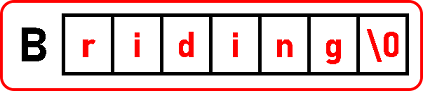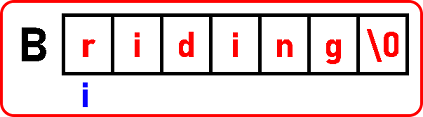# How to Find Duplicates in a String in C

## How to Find Duplicates in a String in C Language with Examples

In this article, I am going to discuss How to Find Duplicates in a String in C Language with Examples. Please read our previous article where we discussed How to Compare String and Checking Palindrome in C Language with Examples.

##### Finding Duplicates in a String:

Here, we will see how to find duplicates in a string. We have to find out are there any duplicate alphabets or are repeating more than one time in a string.For example, in the given string, ‘i’ is repeated more than one time. We have already seen multiple methods for finding duplicate numbers. Now we will see what are the methods for finding duplicate characters in a string. There are 3 methods for finding duplicate elements in a string:

1. Compare with other letters.
2. Using HashTable or counting
3. Using Bits

The 1st method is the same as what we have learned in arrays like taking a number and comparing the rest of the numbers in an array so we will take an alphabet and compare it with the rest of the alphabet in a string. The method we have already seen so we will not explain it in detail.

The 2nd method is also similar to the one we have already seen. But there is a little change here so we will explain this one.

Then the 3rd one is the new one that is bits which we will cover in next article.

##### Method1: Finding Duplicates in a String by Comparing with other letters

So let us start with the 1st method comparing with other elements.Let’s scan the list from the left-hand side. If so, we have to count it so we can take the help of the ‘j’ pointer and start checking for the count.We have scanned through the rest of the characters in this string and ‘r’ is not found. Next, we will move to the next character and start checking for that. There are two occurrences of ‘i’. We have to stop when we reached ‘\0’ or null character. So, this is the 1st method to find duplicates in an array. And we have also done the analysis for that and the time taken is in order of n2.

##### Program for Finding Duplicates in a String using C language:
#include <stdio.h>
#include <stdlib.h>
int main ()
{
char B[] = “riding”;
printf (“String is \”%s\”\n”, B);
for (int i = 0; i <= 4; i++)
{
int count = 1;
for (int j = i + 1; B[j] != ‘\0’; j++)
{
if (B[i] == B[j])
{
count++;
B[j] = -1;
}
}
if (count > 1 && B[i] != -1)
{
printf (“\’%c\’ is appearing: %d times\n”, B[i], count);
}
}
return 0;
}

Output:##### Method2: Finding Duplicates by Using Hash Table or Counting

Now let us go to the 2nd method which is using a hash table and counting the occurrences of alphabets. For using a hash table, we should have an array to work as a hash table. What should be the size of the array? It depends on the numbers that we are storing. So, whatever the largest number that we are storing we need an array of that much size.

We are going to store alphabets and these alphabets are having their ASCII codes. I have taken all these alphabets as a lower key so that they are in the range of lowercase alphabets.

If you know the range of lower case that starts from 97 to 122. So, it means we need an array up to the maximum size of 122 and I will start using that area from 97 onwards means all the elements from 0 to 96 are useless.

Why do create that much size of the array when we know that the first lower case is 97 and the last is 122. So why can’t we call ‘a’ as a zero? And the ‘b’ as one and so on. It means an area of a size twenty-five will be sufficient for us. So, this is how we can reduce the size of the hash table. We can take just of size twenty-six.

And we can find out the counting of these alphabets and duplicates alphabets and minder. This is only for lowercase alphabets. There are no upper cases so let us draw in a hash table and run the procedure and see how we can count them.So here I have an array for the hash table that starting index is 0 and the ending index is 25. Now let us start the procedure and scan for the string. The first alphabet in our string is ‘r’ and ASCII for ‘r’ is 114. So, 114 – 97 = 17. So go to index 17 and increment it in the above hashTable.Then move to the next alphabet which is ‘i’. ASCII code for ‘i’ is 105. So, 105 – 97 = 8. So go to index 8 and increment it in hashTable.Now move to the next alphabet which is ‘d’. ASCII code is 100. So, 100 – 97 = 3. So go to index 3 and increment it in hashTable.Then move to the next alphabet which is ‘i’. ASCII code for ‘i’ is 105. So, 105 – 97 = 8. So again, go to index 8 and increment it.Now move to the next alphabet which is ‘n’. ASCII code is 110. So, 110 – 97 = 13. So go to index 13 and increment it in hashTable.Now move to the next alphabet which is ‘g’. ASCII code is 103. So, 103 – 97 = 6. So go to index 6 and increment it in hashTable.We have to stop when we reached the null character or ‘\0’. Now we have the counting of all the alphabets in our Hash Table. The first index of the hash table means 0 it means 0 + 97. Then 1 means 1 + 97 = 98 and so on. Because we have subtracted 97, now add 97 to all indices. So that’s all we can get back the alphabets by adding 97 into the indices in the hash table. So let us write full code here to perform the same procedure to display only those alphabets which are appearing more than one time.

##### Program for Finding Duplicates by Using Hash Table in C Language:
#include <stdio.h>
#include <stdlib.h>
int main ()
{
char B[] = “riding”;
int H;
printf (“String is \”%s\”\n”, B);
for (int i = 0; i < 26; i++)
{
H[i] = 0;
}
for (int i = 0; B[i] != ‘\0’; i++)
{
H[B[i] – 97] += 1;
}
for (int i = 0; i < 26; i++)
{
if (H[i] > 1)
{
printf (“\’%c\’ is repeating”, i + 97);
printf (“: %d times\n”, H[i]);
}
}
return 0;
}
###### Output:We have shown only lower case alphabets. Not if there are uppercase also or upper and lower cases are mixed. Then you have to increase the size of the hash table. Now there is one more method that is using bits and we will explain this in the next article.